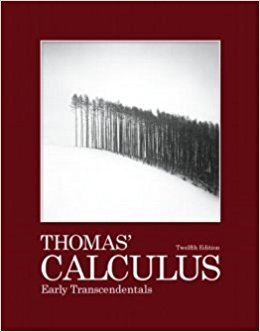×

×

# Solutions for Chapter 12: Vectors and the Geometry of Space## Full solutions for Thomas' Calculus Early Transcendentals | 12th Edition

ISBN: 9780321588760Solutions for Chapter 12: Vectors and the Geometry of Space

Solutions for Chapter 12
4 5 0 331 Reviews
20
2
##### ISBN: 9780321588760

Thomas' Calculus Early Transcendentals was written by and is associated to the ISBN: 9780321588760. This textbook survival guide was created for the textbook: Thomas' Calculus Early Transcendentals, edition: 12. This expansive textbook survival guide covers the following chapters and their solutions. Chapter 12: Vectors and the Geometry of Space includes 76 full step-by-step solutions. Since 76 problems in chapter 12: Vectors and the Geometry of Space have been answered, more than 32979 students have viewed full step-by-step solutions from this chapter.

Key Calculus Terms and definitions covered in this textbook
• Continuous at x = a

lim x:a x a ƒ(x) = ƒ(a)

• Distance (in Cartesian space)

The distance d(P, Q) between and P(x, y, z) and Q(x, y, z) or d(P, Q) ((x ) - x 2)2 + (y1 - y2)2 + (z 1 - z 2)2

• Distance (on a number line)

The distance between real numbers a and b, or |a - b|

• Frequency table (in statistics)

A table showing frequencies.

• Future value of an annuity

The net amount of money returned from an annuity.

• Hypotenuse

Side opposite the right angle in a right triangle.

• Integrable over [a, b] Lba

ƒ1x2 dx exists.

• Linear correlation

A scatter plot with points clustered along a line. Correlation is positive if the slope is positive and negative if the slope is negative

• Linear regression

A procedure for finding the straight line that is the best fit for the data

• NDER ƒ(a)

See Numerical derivative of ƒ at x = a.

• Polar coordinates

The numbers (r, ?) that determine a point’s location in a polar coordinate system. The number r is the directed distance and ? is the directed angle

• Positive association

A relationship between two variables in which higher values of one variable are generally associated with higher values of the other variable, p. 717.

• Probability distribution

The collection of probabilities of outcomes in a sample space assigned by a probability function.

• Quotient rule of logarithms

logb a R S b = logb R - logb S, R > 0, S > 0

• Replication

The principle of experimental design that minimizes the effects of chance variation by repeating the experiment multiple times.

• Terminal point

See Arrow.

• Terms of a sequence

The range elements of a sequence.

• Third quartile

See Quartile.

• Trichotomy property

For real numbers a and b, exactly one of the following is true: a < b, a = b , or a > b.

• Vertical line

x = a.